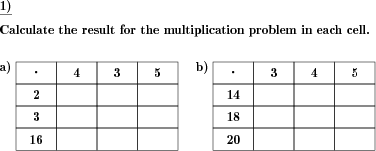Custom math worksheets at your fingertipsDetails for problem "Table of multiplication problems"

Quickname: 5545

Elementary School, Primary School, Junior High School, Middle School, High School, Senior High School.

Summary

Matrix of multiplication problems with whole numbers

ExampleDescription

Times tables need a lot of practice to achieve fluency and this worksheet provides a variety to practice. Several multiplication problems are presented in the form of a matrix. All numbers are whole numbers, and may be positive or negative only, or mixed. The complexity of problems can be selected as per grade level from third-grade to tenth-grade, to be used as a "do now" bell-ringer activity, and group work. This math worksheet would also be perfect for a quick assessment tool. By completing this worksheet successfully, the following milestones can be achieved.

1. Revise all times table learned.
2. Develop strong concepts and fluency of the times table.
3. Build up the times tables skills for future mathematical studies.
4. Build real-life skills based on multiplication.
5. Introduce the concept of matrix especially in junior grades.

Several variations are possible to generate these worksheets as per the grade or requirement of the learner.

• The numbers and results will all be within a choosable number range.
• The size of the matrix can be selected from 1x1 up to 4x4 cells in size, containing from nine up to sixteen individual multiplication problems.
• The number of matrices can be selected.

The answer key consists of filled values in the matrix.

Download free printable worksheets for this math problem here. The worksheet contains the problems only, the solution sheet includes the answers. Just click on the respective link.

•Worksheet 1Solution sheet with answers
•Worksheet 2Solution sheet with answers
•Worksheet 3Solution sheet with answers

If you can not see the solution sheets for download, they may be filtered out by an ad blocker that you may have installed. If this is the case, please allow ads for this page and reload the page. The solution sheets will then reappear.

• Do these sample worksheets do not really fit?
• Do you need more math worksheets, with a different level of difficulty?
• Would you like to combine different problems on a worksheet and adjust them to your needs?
• As a teacher, you can put together your own worksheets using the automatically generated math problems provided.
With a free initial credit, you can start creating your own math worksheets in a few minutes.

You can try it for free! Register here, to create custom worksheets now!

Customization options for this problem

Parameter
Possible values
Number of tables
1, 2, 3, 4
Width
1, 2, 3, 4
Height
1, 2, 3, 4
Number range
100, 200, 500, 1000, 10000, 100000
Positive/Negative
mixed, positive, negativeDeutsche Version dieser Aufgabe
These informational pages with samples describe math problems that can be combined on custom math worksheets with solutions for home and K-12 school use.
Deutsche Seiten
×